# Besicovitch almost-periodic functions

(diff) ← Older revision | Latest revision (diff) | Newer revision → (diff)

A class of almost-periodic functions in which the analogue of the Riesz–Fischer theorem is valid: Any trigonometric serieswhere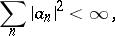is the Fourier series of some-almost-periodic function. The definition of these functions ,  is based on a generalization of the concept of an almost-period, and certain additional ideas must be introduced in it. A set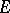of real numbers is called sufficiently homogeneous if there exists an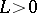such that the ratio between the largest number of members ofin an interval of length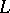and the smallest number of members in an interval of the same lengthis less than 2. A sufficiently homogeneous set is also relatively dense. A complex-valued function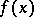,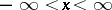, summable to degreeon any finite interval of the real axis, is called a Besicovitch almost-periodic function if to eachthere corresponds a sufficiently homogeneous set of numbers (the so-called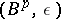-almost-periods of):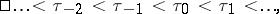such that for each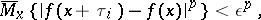and for each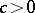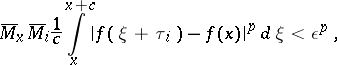where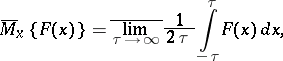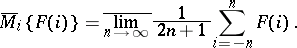Hereis a real-valued function, defined, respectively, for a real variable and an integer argument.

How to Cite This Entry:
Besicovitch almost-periodic functions. Encyclopedia of Mathematics. URL: http://encyclopediaofmath.org/index.php?title=Besicovitch_almost-periodic_functions&oldid=11650
This article was adapted from an original article by E.A. Bredikhina (originator), which appeared in Encyclopedia of Mathematics - ISBN 1402006098. See original article